# Mathematica入门–Function Definitions(函数定义)

• A+

## 函数定义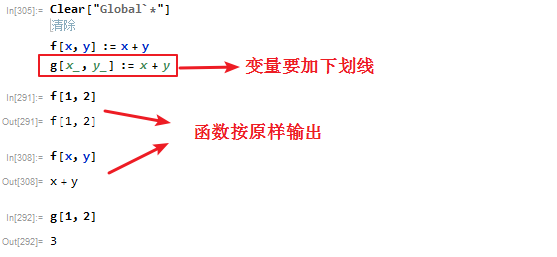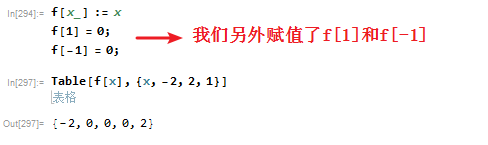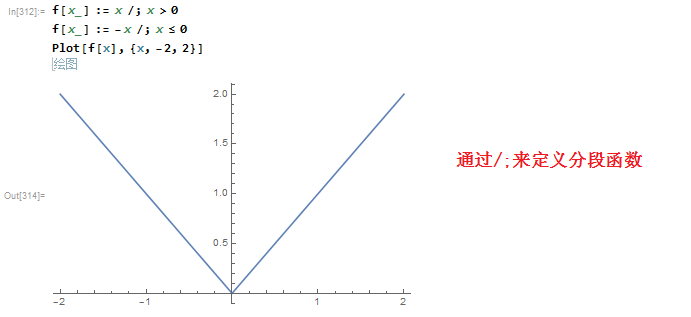``````def f(x,y):
return x+y``````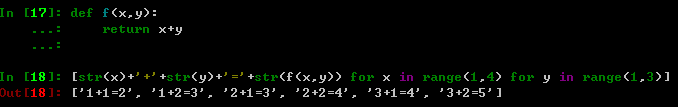``````def f(x):
if x>=0:
return x
else:
return -x``````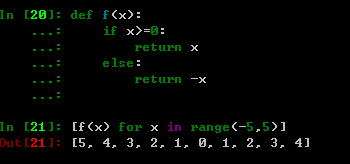• 微信公众号
• 关注微信公众号
•• QQ群
• 我们的QQ群号
•